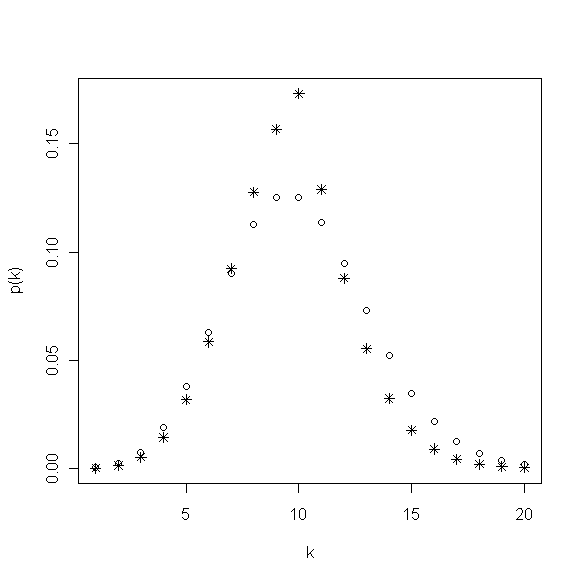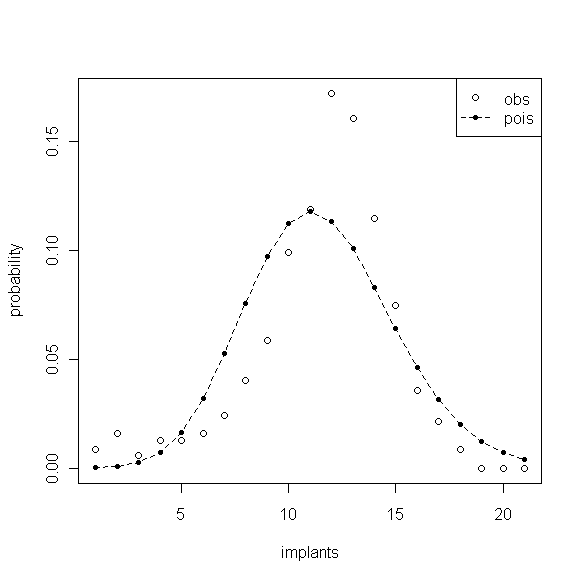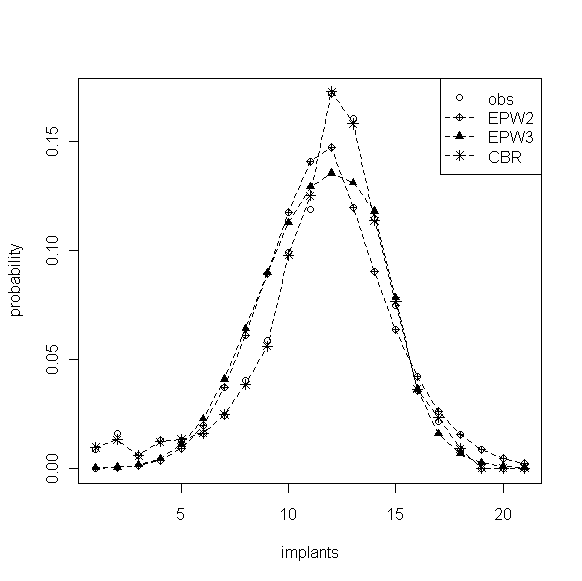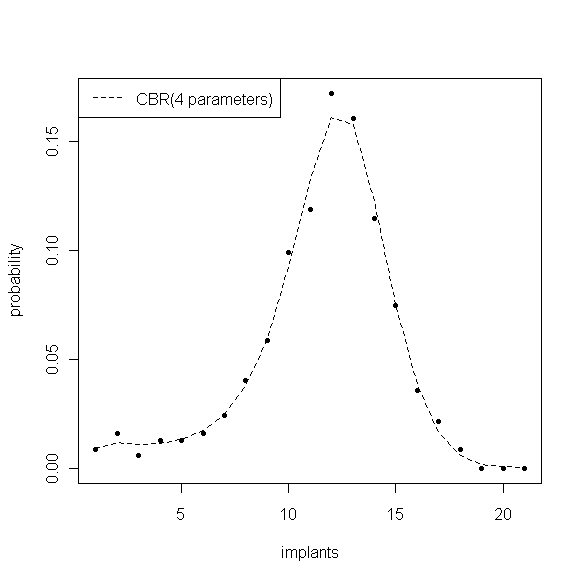# 二 两种泊松低方差问题的处理方法

## 1. 加权泊松分布

Rao CR (1965)提出，若随机变量Y服从加权泊松分布，其密度函数为

$$P(Y=k)=\frac{e^{-\lambda}\lambda^k\omega_k }{Wk!}, k=0,1, \ldots ; \lambda>0$$

\begin{equation} W=\sum^{\infty}_{k=1}\frac{e^{-\lambda}\lambda^k\omega_k }{k!}\label{a} \end{equation}

$$\omega_k=\left( \begin{array}{ll} e^{\beta_1(\lambda-k)} & ,k\leq\lambda\\e^{\beta_2(\lambda-k)} & ,k>\lambda\\\end{array} \right)$$## 2. 纯生过程模型（CBR）

$$P\left(X(t+\sigma)=n+1|X(t)=n\right)=\lambda_n\sigma+o(\sigma)$$

$$(p_1(1),p_2(1),\ldots,p_n(1))=(1,0,0,\cdots,0)exp(Q)$$

$$p_i(1)=P\left(X(1)=i\right)$$

$$exp(Q)=e^Q=E+\frac{Q}{1}+\frac{Q^2}{2!}+\frac{Q^3}{3!}+\cdots$$

$$Q=\left( \begin{array}{ccc} -\lambda_1 & \lambda_1 & 0 & \ldots & 0\\0 &-\lambda_2 & \lambda_2 & \ldots & 0\\0 & 0 & -\lambda_3 & \ldots & \vdots\\ \vdots & \vdots & \vdots & \ddots & \lambda_{n-1}\\ 0 & 0 & 0 & \ldots & -\lambda_n\\\end{array} \right)$$

$$p'(t)=Qp(t); p(t)=ce^{Qt}; p(1)=ce^Q;$$

$$p(0)=c; p(1)=p_0e^Q; p(0)=(1,0,0,\ldots,0)$$

# 三、参数估计

\begin{equation} -LnL(\lambda,\beta_1,\beta_2)=n[\lambda-\bar{x}ln\lambda+lnW]+\beta_1\sum^{[\lambda]}_{k=x_{min}}(\lambda-k)f_k+<br /> \beta_2\sum^{x_{max}}_{k=[\lambda]+1}(k-\lambda)f_k \label{b} \end{equation}

$$-LnL(\lambda_1,\lambda_2,\ldots,\lambda_N)=\sum^N_{k=1}f_klnp_k(1)$$

# 四、数据实例## 1. 加权泊松分布## 2. CBR分布

CBR参数的极大似然估计$$\hat{\lambda}_1,\hat{\lambda}_2,\ldots,\hat{\lambda}_{21}$$= {4.66, 7.90, 22.97, 15.02, 18.23, 19.18, 16.49, 14.40, 13.15, 10.01, 9.33, 6.90, 6.32, 6.26, 5.40, 6.28, 3.27, 0.00, 2.00, 2.00, 1.00}，图3中显示的 CBR 拟合效果非常好，几乎与经验分布重合。但模型中含有过多的参数使得估计精度大大降低，其中$$\hat{\lambda}_3$$的估计标准误为12.59，置信区间宽近50。虽然对于拥有多个事件发生率$$\hat{\lambda}_k$$的 CBR 分布能够灵活地刻画事件发生数之间的任何概率变化，但参数时过多模型的过度拟合是没有太大意义的，也不易于控制和分析。如前所述，可以利用该模型的$$\lambda$$建立关于k的函数，从而减少模型中的参数个数，在该例子中事件数分类较多时这种做法就显得十分必要。Faddy(2001) 就从众多函数形式中找到了一种能很好地模拟事件数发生概率在最初增长较快而后缓慢下降特点的四参数模型：

$$\lambda_k=a(k^be^{-ck}+d), k\geq1$$## 3. 组别分析

$$\Lambda(x)=\frac{sup{L(\theta|x):\theta\in\Theta_o}}{sup{L(\theta|x):\theta\in\Theta}}$$

$$-2log(\Lambda)$$ 自由度r p值

EWP2 4.789 2 0.0912
EWP3 11.980 3 0.0075
CBR 10.764 4 0.0294